# DAV Class 6 Maths Chapter 5 Worksheet 4 Solutions

The DAV Class 6 Maths Solutions and DAV Class 6 Maths Chapter 5 Worksheet 4 Solutions of Percentage and its Applications offer comprehensive answers to textbook questions.

## DAV Class 6 Maths Ch 5 WS 4 Solutions

Question 1.
Fill in the following blanks:

(a) Principal = ₹ 600, Time = 2 years, Rate of interest = 10% p.a., simple interest = _____.
Solution:
Here, P = ₹ 600,
T = 2 years,
R = 10% p.a.
∴ Simple interest, S.I. = $$\frac{\mathrm{P} \times \mathrm{R} \times \mathrm{T}}{100}$$
=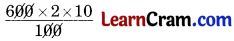= ₹ 120
Hence, the required S.I. = ₹ 120.(b) Principal = ₹ 6500, Time = 1½ years, Rate of interest = 10% p.a., simple interest = _____.
Solution:
Here, P = ₹ 6500,
T = 1 $$\frac{1}{2}$$ years
= $$\frac{3}{2}$$ years,
R = 10 % p.a.
∴ Simple interest, S.I. = $$\frac{\mathrm{P} \times \mathrm{R} \times \mathrm{T}}{100}$$
=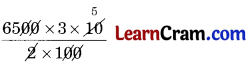= ₹ 975
Hence, the required Simple interest S.I. = ₹ 975.

(c) Principal = ₹ 1280, Time = 2½ years, Rate of intere.st = 2% %, Simple interest = _____, Amount = _____.
Solution:
Here, P = ₹ 1280,
T = 2 $$\frac{1}{2}$$ years
= $$\frac{5}{2}$$ years,
R = 2 $$\frac{3}{4}$$ %
= $$\frac{11}{4}$$ % p.a.
∴ Simple interest, S.I. = $$\frac{\mathrm{P} \times \mathrm{R} \times \mathrm{T}}{100}$$
=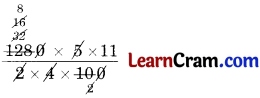= ₹ 88
Amount = P + S.I.
= ₹ 1280 + ₹ 88
= ₹ 1368
Amount = ₹ 1368.

(d) Principal = ₹ 2600, time = 3 years, Rate of interest = 5½ % p.a. Simple interest = _____ , Amount = ____ .
Solution:
Here, P = ₹ 2600,
T = 3 years
R = 5 $$\frac{1}{2}$$ %
= $$\frac{11}{2}$$ % p.a.
∴ Simple interest, S.I. = $$\frac{\mathrm{P} \times \mathrm{R} \times \mathrm{T}}{100}$$
=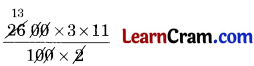= ₹ 429

Amount = P + S.I.
= ₹ 2600 + ₹ 429
= ₹ 3029
Amount = ₹ 3029.Question 2.
Hari borrowed ₹ 450 from his friend at 6% per annum. Fie returned the amount after 8 months. How much money did he pay?
Solution:
Here, P = ₹ 450,
R = 6% p.a.,
T = 8 months
= $$\frac{8}{12}=\frac{2}{3}$$ years
∴ Simple interest, S.I. = $$\frac{\mathrm{P} \times \mathrm{R} \times \mathrm{T}}{100}$$
== ₹ 18

∴ Amount = P + S.I.
= ₹ 450 + ₹ 18
= ₹ 468
Hence, the money to be returned = ₹ 468.

Question 3.
Find the simple interest on ₹ 2000 for 6 months at the rate of 4 % per annum.
Solution:
Here, P = ₹ 2000,
R = 4 $$\frac{1}{2}$$% p.a.
= $$\frac{9}{2}$$ % p.a.,
T = 6 months
= $$\frac{6}{12}=\frac{1}{2}$$ years
∴ Simple interest, S.I. = $$\frac{\mathrm{P} \times \mathrm{R} \times \mathrm{T}}{100}$$
== ₹ 45
Hence, the S.I. = ₹ 45.Question 4.
A farmer borrowed ₹ 5300 at 8% p.a. At the end of 2 years, he cleared his account by paying ₹ 4600 and watch.
Find the cost of watch.
Solution:
Here, P = ₹ 5300,
R = 8% p.a.,
T = 2$$\frac{1}{2}$$ years
= $$\frac{5}{2}$$ years Z Z
∴ Simple interest, S.I. = $$\frac{\mathrm{P} \times \mathrm{R} \times \mathrm{T}}{100}$$
== ₹ 1060

Amount = P + S.I.
= ₹ 5300 + ₹ 1060
= ₹ 6360
Cost of watch = ₹ 6360 – ₹ 4600 = ₹ 1760
Hence, the required cost = ₹ 1760.

Question 5.
Harish borrows ₹ 2500 at 3% p.a. and ₹ 1000 at 5% p.a. interest. What interest will Harish have to pay after 2 years?
Solution:
Case I:
Here, P = ₹ 2500,
R = 3% p.a.,
T = 2 years
∴ Simple interest, S.I. = $$\frac{\mathrm{P} \times \mathrm{R} \times \mathrm{T}}{100}$$
=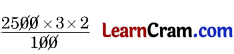= ₹ 150

Case II:
Here, P = ₹ 1000,
R = 5% p.a.,
T = 2 years
∴ Simple interest, S.I. = $$\frac{\mathrm{P} \times \mathrm{R} \times \mathrm{T}}{100}$$
=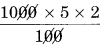= ₹ 100

Total simple interest = ₹ 150 + ₹ 100 = ₹ 250
Hence, the required simple interest = ₹ 250.Question 6.
Rohan deposited ₹ 5000 at 8% p.a. for 3 $$\frac{1}{2}$$ years and Sunil deposited ₹ 5000 at 6% p.a. for 4 years. Who will get more interest? What amount will each get?
Solution;
Case I:
Here, P = ₹ 5000,
R = 8% p.a.,
T = 3 $$\frac{1}{2}$$
= $$\frac{7}{2}$$ years
∴ Simple interest, S.I. = $$\frac{\mathrm{P} \times \mathrm{R} \times \mathrm{T}}{100}$$
=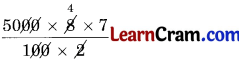= ₹ 1400

Case II:
Here, P = ₹ 5000,
R = 6% p.a.,
T = 4 years
∴ Simple interest, S.I. = $$\frac{\mathrm{P} \times \mathrm{R} \times \mathrm{T}}{100}$$
=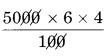= ₹ 1200

Amount = P + S.I.
= ₹ 5000 + ₹ 1200
= ₹ 6200

∴ Rohan gets S.I. = ₹ 1400
and Sunil gets S.I. = ₹ 1200
∴ Rohan gets more S.I.
Amount got by Rohan = ₹ 6400
and by Sunil = ₹ 6200
So, Rohan gets more amount.